## Introduction

Various studies have been published on electric-field-controlled magnetic effects in recent years, including magnetic domain wall propagation,1, 2, 3, 4, 5, 6, 7, 8 magnetic phase transitions,9, 10, 11, 12 spin polarization,13, 14 magnetic anisotropy15, 16, 17, 18, 19, 20, 21, 22, 23, 24, 25, 26, 27, 28, 29, 30, 31, 32 and exchange bias.33, 34, 35, 36, 37 Electric-field control of perpendicular magnetic anisotropy (PMA) would open up new prospects for the realization of high-density magnetic memory and logic technologies operating at low energy consumption levels. Attempts to attain this goal have mostly focused on charge accumulation or band shifting in ultrathin ferromagnetic layers with a metal oxide gate dielectric.15, 16, 17, 18, 19, 20 In these systems, stable reversal of perpendicular magnetization can be realized when precessional motion of magnetization is triggered by short voltage pulses.19, 20 Giant modulations of PMA have also been obtained by voltage control of oxygen ion migration in metal/metal oxide bilayers.32 Another popular approach towards the engineering of electric-field-controlled magnetic properties is based on interfacial mechanical strain coupling between ferromagnetic and ferroelectric materials in multiferroic hybrids.4, 5, 6, 8, 10, 12, 21, 22, 23, 24, 25, 26, 27, 28, 29, 30, 31, 38 In this case, transfer of lattice strains across interfaces alters the magnetoelastic anisotropy of a ferromagnetic layer via inverse magnetostriction. Anisotropy modulations of more than one order of magnitude have been obtained, which has enabled full electric-field control of the magnetization orientation in two-phase multiferroic systems. However, in spite of intense research efforts in recent years, efficient electric-field control via strain coupling has thus far been limited to ferromagnetic films with in-plane magnetization. Although small modulations of PMA have been shown21, 27, 28, 29 and reversal of local magnetic areas has been demonstrated,30 fully deterministic and reversible electric-field-induced switching between perpendicular and in-plane magnetization states in uniform films has remained elusive despite its technological relevance.

Here, we report on purely electric-field-driven 90° magnetization switching in PMA-Cu/Ni multilayers on BaTiO3 at room temperature. Magnetization rotation in our strain-coupled system is fully reversible and repeatable. Also, we demonstrate that 180° magnetization reversal is obtained in an electric field when the out-of-plane symmetry of PMA is temporarily broken by a small magnetic field. Electric-field-induced magnetic switching is driven by large variations of magnetic anisotropy in the Cu/Ni multilayer originating from ferroelastic domain switching in BaTiO3. The electric field that is required to reorient the magnetization is modest and strict limitations on the total multilayer thickness are not imposed. Our results do therefore present a new and promising realization of fully electric-field-controlled magnetization switching in a PMA system.

## Materials and methods

### Multilayer growth

[Cu(9 nm)/Ni(2 nm)]5/Cu(9 nm) multilayers were grown by molecular beam epitaxy on nominally in-plane -poled BaTiO3 single-crystal substrates in an ultrahigh vacuum chamber with a base pressure below 1010 Torr.39 Before Cu and Ni deposition, a 1-nm thin Fe buffer layer was grown at 300 °C. All Cu and Ni layers were deposited at room temperature and the multilayers were covered by 5 nm of Au to prevent oxidation during sample characterization. The growth process was monitored by reflection high-energy electron diffraction (RHEED) and detailed structural analysis was performed using an X-ray diffraction (XRD) measurement system with Cu Kα radiation.

### Magnetic and ferroelectric characterization

Electric-field-induced magnetic switching in the Cu/Ni multilayers was studied using the magneto-optical Kerr effect (MOKE). A laser-based MOKE setup with polar and longitudinal measurement geometries and a laser spot diameter of about 500 μm was used to record magnetic hysteresis curves in an out-of-plane electric field of up to ±10 kV cm−1. Time-resolved MOKE measurements were conducted using a digital oscilloscope (WavePro 7100A, Teledyne LeCroy, NY, USA) with a sampling rate of 20 GS s−1 and a bandwidth of 1 GHz. MOKE microscopy was performed to visualize the evolution of the magnetic domain structure in an applied electric field and to measure local magnetic hysteresis curves in polar Kerr geometry. Polarization switching in the BaTiO3 substrate was measured using a ferroelectric materials tester (TF Analyzer 2000, aixACCT Systems, Aachen, Germany) and differential interference contrast microscopy.

## Results

### Structural characterization

Figure 1a shows reflection high-energy electron diffraction patterns of the top Ni and Cu layers of a [Cu(9 nm)/Ni(2 nm)]5/Cu(9 nm)/Fe(1 nm)/BaTiO3(001) multiferroic heterostructure. The high-energy electron diffraction patterns confirm fully epitaxial film growth with an in-plane orientation of Ni || Cu || Fe || BaTiO3. The X-ray diffraction spectrum of Figure 1b confirms that secondary phases are absent in the Cu/Ni multilayer. The BaTiO3 substrate exhibits a tetragonal crystal structure at room temperature with lattice constants aBTO=3.992 Å and cBTO=4.036 Å and a ferroelectric polarization aligned along the c axis. The splitting of the BaTiO3 reflections into (h00) and (00l) peaks signifies the coexistence of two types of ferroelectric domains. Domains with in-plane ferroelectric polarization (hereafter referred to as a-domains) exhibit a rectangular in-plane lattice structure (x1=aBTO and x2=cBTO) and an out-of-plane lattice spacing of x3=aBTO. The a-domains are separated by domains with a perpendicular-to-plane polarization (c-domains). The in-plane lattice structure of the c-domains is square (x1=x2=aBTO) and x3=cBTO. Since the BaTiO3 (h00) reflections are more intense than the corresponding (00l) peaks, the a-domains are larger than the c-domains in the pristine BaTiO3 substrate. From the integrated intensities of the split peaks, the total sample coverage of a-domains is estimated as 93% in this sample.

Figure 1c shows a clear X-ray diffraction interference fringe from the Cu/Ni multilayer. The pronounced satellite peaks are a signature of high structural quality and smooth interfaces. The Cu/Ni bilayer thickness D can be calculated from the angular positions of the satellite peaks, which are decomposed into Voigt functions40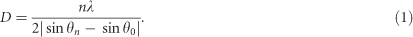Here, θn and θ0 are the diffraction angles of the nth order satellite and the fundamental Bragg peak, and λ is the X-ray wavelength. Fitting of the experimental data using Equation 1 gives D=11.6 nm, which is compatible with the nominal layer thickness of the Cu(9 nm)/Ni(2 nm) bilayer.

The shift of the Cu(002)/Ni(002) reflection from the diffraction angles of bulk Cu(002) (aCu=3.615 Å) and bulk Ni(002) (aNi=3.524 Å) reveals a mean out-of-plane lattice spacing of 3.673 Å. Reciprocal lattice maps around the (002) and (202) diffraction peaks indicate a corresponding in-plane lattice spacing of 3.590 Å for the as-grown Cu/Ni multilayer on BaTiO3. The lattice strain in the Ni layers is thus tensile and amounts to 1.9%. This experimental value is in excellent agreement with a theoretical calculation based on the actual thicknesses of Cu (9 nm) and Ni (2 nm) and their elastic stiffness, which yields an in-plane lattice constant of 3.592 Å for a coherent free-standing Cu/Ni multilayer. We note that the reciprocal lattice map around the (202) diffraction peak shown in Figure 1d clearly shows that the misfit strain caused by the lattice mismatch between the Ni/Cu multilayer and BaTiO3 substrate is relaxed in the epitaxially grown film. A detailed theoretical analysis of strain and magnetic anisotropy is given after the next section.

### Electric-field-driven 90° magnetization switching

Cu/Ni multilayers were selected for this study because they provide strong PMA in a relatively large Ni thickness range (typically 1.5 nm<tf <8 nm).41 Moreover, since PMA in Cu/Ni originates from a magnetoelastic effect, it is predicted to be sensitive to electric-field-induced strain modulations.38 Figure 2 shows the experimental configuration, polarization switching in BaTiO3, and normalized out-of-plane and in-plane magnetic hysteresis curves for different electric fields across the BaTiO3 substrate. The data in Figures 2c–f provide macroscopic information about the magnetic configuration at room temperature and were recorded using a MOKE setup with polar and longitudinal measurement geometries. Before applying any electric field (Figure 2c), a square-shaped out-of-plane hysteresis curve is obtained while the in-plane one is slanted and almost completely unopened. These measurements clearly establish that the Cu/Ni multilayer exhibits PMA in the as-grown state. The slope of the in-plane magnetic hysteresis curve corresponds to a magnetic anisotropy strength of Keff ≈ 2.4 × 104 J m−3. When a positive electric field of 10 kV cm−1 is applied to BaTiO3 (Figure 2d), the shapes of both hysteresis curves change instantaneously. Now the out-of-plane curve is slanted and the in-plane hysteresis curve is square. This apparent reversal in the shape of the hysteresis curves indicates a rotation of the easy magnetization axis from perpendicular (E=0 kV cm−1) to in-plane (E=10 kV cm−1), as illustrated in Figure 2a. A very similar result is obtained for a negative electric field of −10 kV cm−1 (Figure 2e). The electric-field-induced magnetic switching effect does therefore not depend on the polarity of the applied bias voltage. Finally, when the electric field is switched off (Figure 2f), the magnetization of the Cu/Ni multilayer rotates back to a perpendicular direction. We note that the electric-field-controlled switching sequence of Figure 2 is fully repeatable over many on/off cycles of applied bias voltage. Moreover, the magnitude of the electric-field-driven effect is very large. The converse magnetoelectric coupling coefficient α=μ0ΔMS/E amounts to 6 × 107 s m−1, which is the highest reported magnetoelectric coupling efficiency for a strain-coupled PMA system. Modest electric-field strengths of ±10 kV cm−1 were used in the switching experiments because it suffices to saturate the polarization of the BaTiO3 substrate in a perpendicular-to-plane direction (Figure 2b and Figure 3).Information about the magnetic microstructure of the Cu/Ni multilayer is provided by MOKE microscopy. The images presented in Figure 3a show the evolution of the magnetic domain structure as a function of out-of-plane electric field. In the initial remanent state (), the sample consists of elongated magnetic stripe domains with alternating perpendicular and mostly in-plane magnetizations. The application of a small electric field of 2 kV cm−1 switches the magnetization into the film plane via fast lateral growth of in-plane magnetized domains (–). The magnetic configuration of does not change upon further increase of the electric-field strength. When the electric field is switched off, perpendicularly magnetized stripe domains are restored (). Reversal of the out-of-plane electric-field direction first enlarges the perpendicular magnetic domains (–), but the process is suddenly reversed at an electric field of −2.5 kV cm−1 (). A further increase of the electric field promotes the growth of domains with in-plane magnetization () until saturation is reached at E=−4 kV cm−1 (). Finally, a magnetic configuration with alternating perpendicular and in-plane domains is again re-established in the Cu/Ni multilayer after the electric field is switched off (). The MOKE microscopy data of Figure 3a indicate that rotation of the easy magnetization axis into the film plane is symmetric in large applied electric fields ( and ), in agreement with the measurements of Figure 2. However, for small electric fields (that is, below saturation) the response can be asymmetric, depending on the direction of the applied electric field relative to the ferroelectric polarization in the BaTiO3 substrate. Another observation relates to the orientation of magnetization inside the in-plane domains. Although the unopened and slanted hysteresis curves in and indicate fully in-plane magnetization in saturation, the hysteresis loops of the same domains are not completely closed in the multidomain state. Magnetic contrast within the domains suggest that the magnetization directions are partly perpendicular, which could be caused by coupling to the perpendicular magnetization of neighboring stripe domains.

The electric-field-controlled switching events and magnetic anisotropy modulations are fully explained by considering the ferroelastic domain pattern of the ferroelectric BaTiO3 substrate and interfacial strain transfer to the Cu/Ni multilayer. Molecular beam epitaxy of the Cu/Ni multilayer onto BaTiO3 produces a fully (001)-oriented layered structure with smooth interfaces. However, owing to the significant differences (>10% ) between the lattice constants of BaTiO3 and those of Cu and Ni, most of the lattice mismatch strain relaxes at the onset of Cu growth. Hereafter, the Cu/Ni multilayer grows with an in-plane lattice constant of 3.590 Å, which corresponds closely to that of a coherent free-standing Cu/Ni multilayer. Thus, a 1.9% tensile strain is induced in the Ni layers, which is determined by the lattice mismatch between Cu and Ni and the elastic stiffnesses and thicknesses of Cu and Ni layers. From this we conclude that the layers are almost completely decoupled from the ferroelastic BaTiO3 domains in the as-grown state. Since the magnetoelastic coefficient of Ni is positive, the tensile strain induces PMA in the Cu/Ni multilayer.If an out-of-plane electric field is applied along the direction of ferroelectric polarization in the c-domains, these domains grow at the expense of a-domains. This ferroelectric switching effect coincides with an abrupt change of the in-plane lattice structure in BaTiO3. In all areas where a-domains are replaced by c-domains, the lattice constant is reduced by 1.1% along one of the crystallographic axes. Since the Cu/Ni multilayer is firmly clamped to the BaTiO3 substrate, a large fraction of this uniaxial compressive strain is transferred to the Cu and Ni layers. This electric-field-controlled strain effect opposes the original growth-induced tensile strain state, leading to magnetization reorientation from a perpendicular-to-plane to an in-plane direction. Figure 3b illustrates the experimental switching sequence of Figure 3a starting with c-domain expansion (). When the ferroelectric substrate is transformed into a single-domain c state, the easy magnetization direction is oriented in the film plane (). The BaTiO3 substrate relaxes back to a polydomain state with ac stripe domains after the electric field is turned off (). In this case, reversible strain transfer to the Cu/Ni multilayer induces PMA on top of the ferroelectric a-domains. When a small electric field is applied against the ferroelectric polarization of the c-domains, the a-domains grow () until the perpendicular polarization in the c-domains abruptly aligns with the external electric field by 180° switching. Afterwards, the c-domains grow at the expense of a-domains () until the single-domain state is reached (). After the electric field is switched off, relaxation into a structure with ac domains commences again (). Throughout this sequence of electric fields, the evolution of the ferroelectric domain structure is mimicked by the domains in the Cu/Ni multilayer because of efficient strain transfer and inverse magnetostriction. We note that the electric field that is required to rotate the magnetization in the MOKE microscopy experiments (Figure 3a) is relatively small compared with the switching field in the polarization hysteresis curve of the BaTiO3 substrate (Figure 2b). This difference could be explained by the time-scale of the two measurements or a sample-to-sample variation. Although the polarization hysteresis curve was obtained at 0.2 Hz, the MOKE microscopy data were typically recorded some 10 s after the electric field was set. We would also like to point out that the initial state in Figure 3a, () was obtained after the application of an electric field and prolonged domain relaxation. Hence, it does not represent the as-grown state. Directly after growth, no domain correlations between the ferroelectric BaTiO3 substrate and the Cu/Ni multilayer exist because of strong relaxation of lattice mismatch strain in the first Cu layer. As a result, as-grown samples exhibit uniform PMA irrespective of the underlying ferroelectric domain pattern.

### Theoretical analysis of strain and magnetic anisotropy

To confirm that interfacial strain transfer from ferroelastic domains in BaTiO3 to the Cu/Ni multilayer explains the experimental results, we analyze the magnetization-dependent part of the free energy of a strained Ni layer written as a function of the direction cosines mi (i=1, 2, 3) of the unit vector m=M/Ms. In our formulation, the interfacial magnetic anisotropy is incorporated into the volumetric energy density ΔF(mi) via the term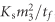, where tf is the Ni layer thickness and Ks is the parameter characterizing the sum of specific energies of two Ni/Cu interfaces. In the crystallographic reference frame with the x3 axis orthogonal to the interfaces, the resulting energy density ΔF(mi) can be written as42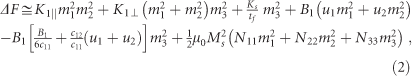where K1||(tf) and K1(tf) are the in-plane and out-of-plane magnetocrystalline anisotropy constants of fourth order,43 μ0 is the permeability of vacuum, Nii are the diagonal components of the tensor of demagnetizing factors, u1 and u2 are the in-plane normal strains (shear strains are absent in our case), B1 is the magnetoelastic coefficient44 and c11 and c12 are the elastic stiffnesses at fixed M (we use the Voigt matrix notation for strains and elastic constants). Using the relations m1=cosφ sinθ, m2=sinφ sinθ and m3=cosθ, we express the energy density ΔF through the polar angle θ and the azimuth angle φ of the magnetization direction. Numerical minimization of the function ΔF(φ,θ) allows us to determine the equilibrium magnetization orientations in the cubic Ni layers for different in-plane lattice strains u1 and u2. We performed this minimization for Ni films with tf=2 nm and large in-plane dimensions ensuring N11=N22=0 and N33=1 using the following values of the involved material parameters: Ms=4.85 × 105 A m−1,44 Ks=4.3 × 10−4 J m−2,45 K1||=−2.5 × 104 J m−3, K1=−1.2 × 104 J m−3,43 B1=9.2 × 106 J m−3,46 c11=2.465 × 1011 J m−2 and c12=1.473 × 1011 J m−2.47 In the free-standing multilayer, the lattice matching between the 2-nm thick Ni layers and the 9-nm thick Cu films produces tensile strains u1=u2 1.9% in Ni. (This theoretical value is smaller than the nominal misfit strain of 2.58% because Cu layers are slightly compressively strained by Ni ones in elastic equilibrium.) Since the magnetoelastic coefficient B1 is positive, these strains stabilize a perpendicular magnetization orientation in the Ni layers (θ=0 or 180°). The mechanical interaction between the Ni/Cu multilayer and a thick BaTiO3 substrate creates additional strains δu1 and δu2 in the Ni layers. Owing to significant differences between the lattice constants of BaTiO3 and those of Cu, the nominal values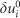of these tensile strains appear to be very big (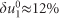and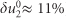). The actual substrate-induced strains, however, are much smaller because of effective strain relaxation during the Cu/Ni multilayer growth. This effect may be described by the relations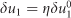and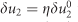with η<<1. Since the strains δu1 and δu2 are tensile, the magnetization of the Ni/Cu multilayer grown on BaTiO3 initially should have a perpendicular orientation irrespective of the parameter η. For η=0, the model gives aNi 3.592 Å and Keff ≈ 2.5 × 104 J m−3, which are in excellent agreement with the experimental data (aNi=3.590 Å and Keff=2.4 × 104 J m−3).

After electric-field-induced switching from an a-domain to a c-domain, the strain δu1 becomes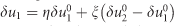. Here, the strain transfer parameter ξ is expected to be much larger than η because of strong interfacial mechanical coupling between the Cu/Ni multilayer and the BaTiO3 substrate during ferroelectric domain switching. Taking η=0 in first approximation, we calculated the equilibrium magnetization orientation after 90° polarization switching in BaTiO3 as a function of the transfer parameter ξ. Figure 4 shows that, at ξ<0.073, the magnetization retains its initial perpendicular direction, whereas at ξ>0.193 it acquires an in-plane orientation (θ=90°). In the intermediate range of 0.073ξ0.193 the Ni layers should have a canted magnetization with the polar angle θ gradually increasing with ξ from 0° to 90°. However, due to efficient transfer of piezoelectric strains, ξ is expected to be close to unity in our experimental system.4 For this scenario the model predicts the formation of two types of magnetic areas in an out-of-plane electric field; one with in-plane magnetization (where the ferroelectric domains underneath have switched from a- to c-type) and another with perpendicular magnetization (where the ferroelectric domains underneath remain the same as during Cu/Ni multilayer growth). This qualitatively agrees with the MOKE microscopy observations in Figure 3. The theoretical model thus fully supports the experimental observations. We note that other magnetoelectric coupling effects, such as electrostatic charge modulation near the BaTiO3 interface, can be excluded as an explanation for electric-field-controlled switching in the Cu/Ni multilayer. First, the symmetric magnetic response in positive and negative electric fields opposes the polarity dependence of charge accumulation. Second, the first Ni layer and the BaTiO3 substrate are separated by a 9-nm thick Cu spacer layer, which exceeds the Thomas–Fermi screening length by more than one order of magnitude.

### Time-resolved measurementsThe dynamics of electric-field-induced magnetic switching was studied by recording the time-resolved MOKE response of strain-coupled Cu/Ni multilayers during the application of a series of voltage pulses across the BaTiO3 substrate. Figure 5a shows the result for five on/off cycles with an electric field of 10 kV cm−1. Initially, the sample consists of alternating domains with perpendicular and partly in-plane magnetizations (similar to and in Figure 3a). In this state, the polar MOKE signal is large. When an out-of-plane electric field is applied, the MOKE response rapidly drops to zero, indicating fast and complete reorientation of the magnetization into the film plane. After the electric field is turned off, the initial magnetization state is re-established. Back and forth switching between the two magnetization states is thus reversible and repeatable. The results of Figure 5a are again explained by ferroelastic domain transformations in BaTiO3. Importantly, they indicate that the time-scale of strain-driven magnetization reorientation is determined by the rate of electric-field induced ac domain switching in BaTiO3, but not by magnetization dynamics itself. Indeed, as can be seen from Figure 5b, the change in polar MOKE signal commences when the electric field reaches ~7 kV cm−1, which closely corresponds to the onset of polarization reversal in BaTiO3 (Figure 2b). Beyond this, the magnetization rapidly reorients into the film plane within ~2 ms. Since the rise time of the electric-field pulse is ~5 ms, the magnetization switching time in this experiment might be limited by the shape of the voltage pulse. Therefore, it should be considered as an upper bound for electric-field-induced magnetic switching in Cu/Ni multilayers. Magnetization reorientation in the off-state (Figure 5a) is not driven by an electric field but rather by ferroelastic domain relaxation in the BaTiO3 substrate, which proceeds more slowly. The small differences in polar MOKE signal after 120 s in the off-state are attributed to minor differences in the ferroelectric a–c domain patterns during subsequent relaxation processes.